# 42 in Binary

Humans communicate with each other through various languages.But have you ever wondered how two machines talk to each other, or how humans communicate with machines?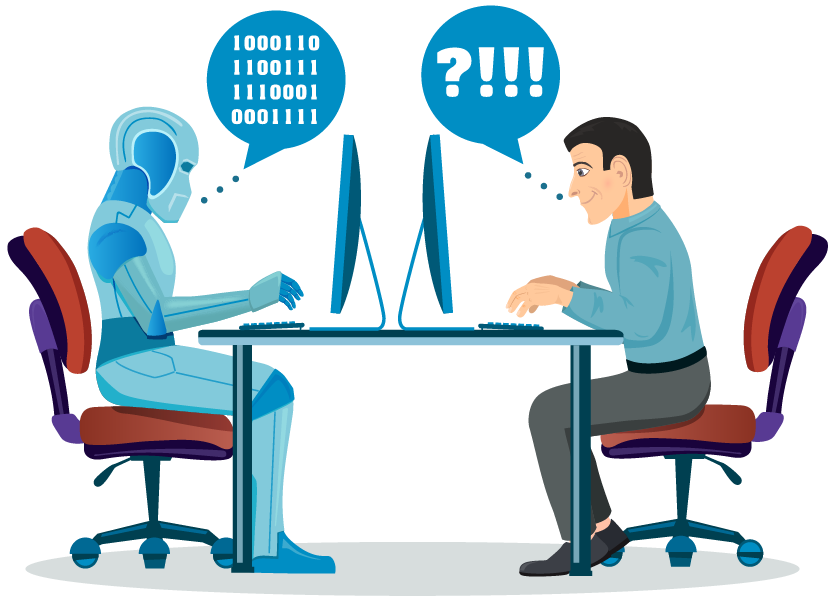Machines can only understand one language which is the binary language.

They convert every form of input from the user to the binary language and then perform the required task.

For example, if you type "Hello World" on your keyboard, your computer first converts this into a binary language, and then it displays your text on the screen.The binary language contains only two numbers, 0 and 1.

These numbers 0 and 1 are called bits.

Let us find an answer to the question 'what is 42 in binary' in this session.

In this mini-lesson, we will learn how to convert 42 to binary by understanding the rules for converting numbers from decimals to binary and how to apply them while solving problems. We will also discover some interesting facts around them.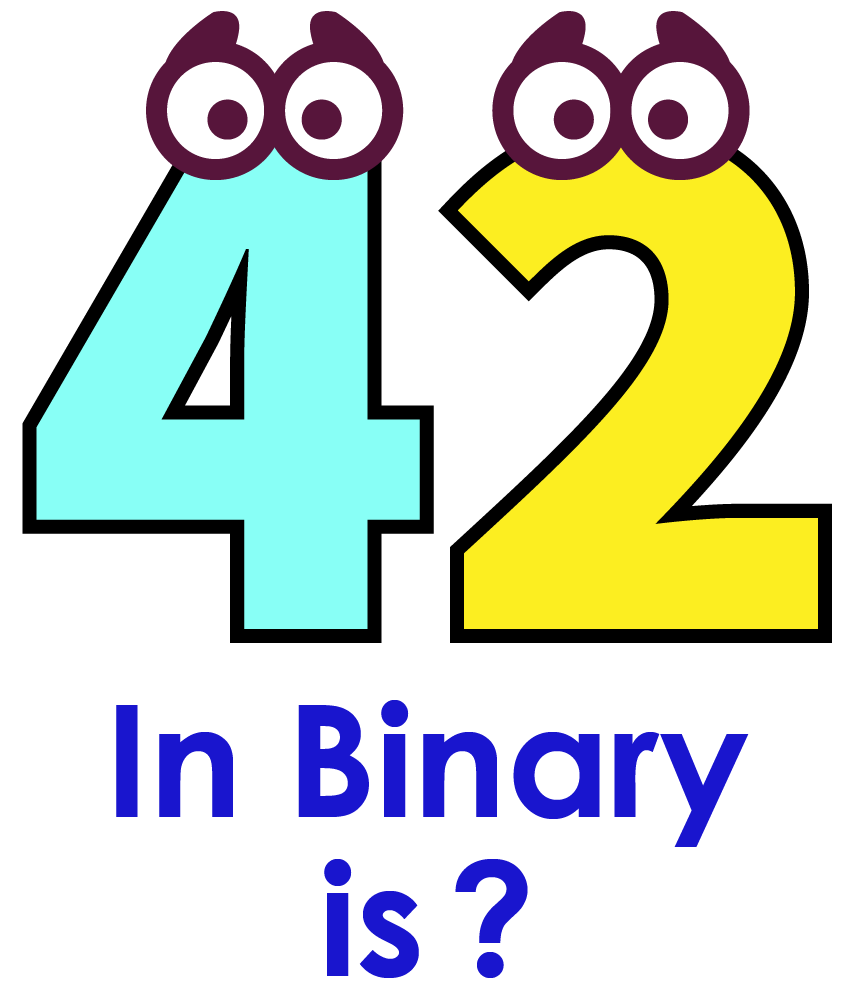## Lesson Plan

 1 What Is Meant By 42 In Binary? 2 Important Notes 3 Solved Examples 4 Challenging Questions 5 Interactive-Questions

## What Is Meant By 42 In Binary?

If someone asks you to write the number 42, you simply write it as 42.

But what if you are asked to write 42 in the decimal form?

$$42_{10}$$ is a decimal representation of 42, where 10 is referred to as the base of the number.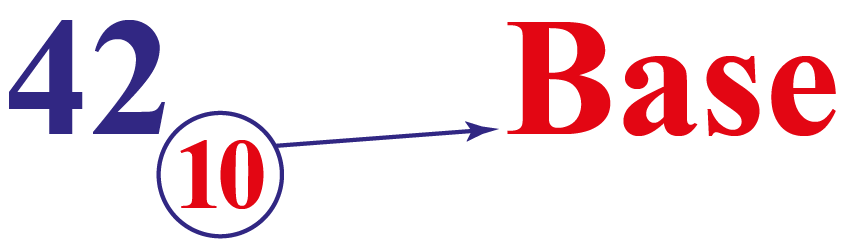Converting 42 from decimal to binary means to write or represent $$42$$ using $$2$$ bits, i.e., $$0$$ and $$1$$.

For converting 42 from decimal to binary, we need to change the base from $$10$$ to $$2$$.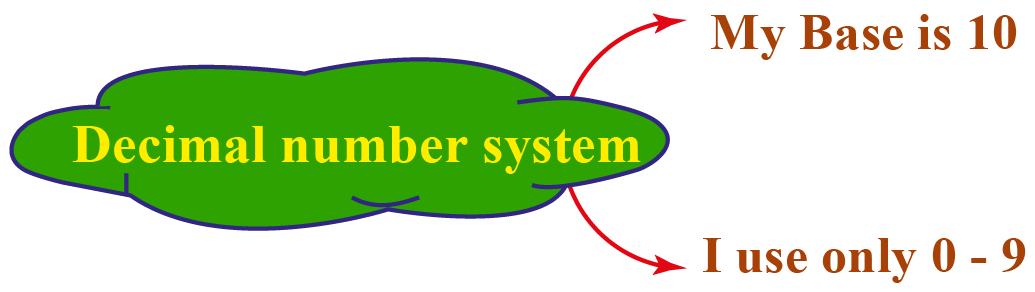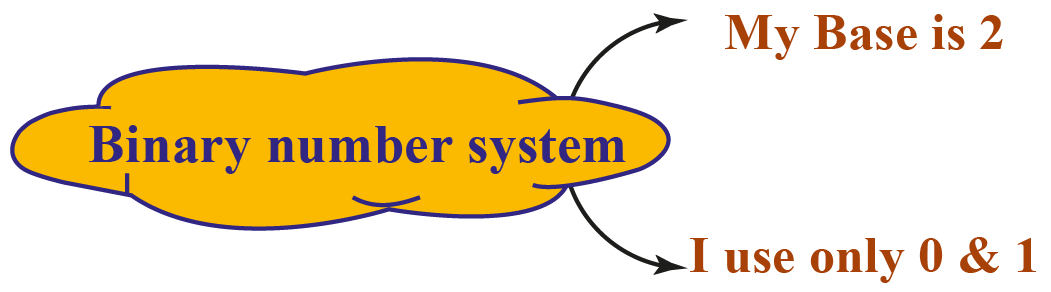$42_{10} \text{ in binary is } 101010_{2}$

43 in Binary

43 in binary is $$101011$$.

13 in Binary

13 in binary is $$1101$$.

47 in Binary

47 in binary is $$101111$$.

## How to Convert 42 From Decimal to Binary?

Let us observe the following steps which show the conversion of 42 from decimal to binary.

Step 1:- Identify the base of the required number. In this case, the base of $$42$$ is $$10$$, i.e., $$42_{10}$$.

Step 2:- Divide the given number 42 by the base (2) and note down the quotient and the remainder in the “quotient-remainder” form.

Repeat this process (dividing the quotient again by the base) until we get the quotient to be less than 2.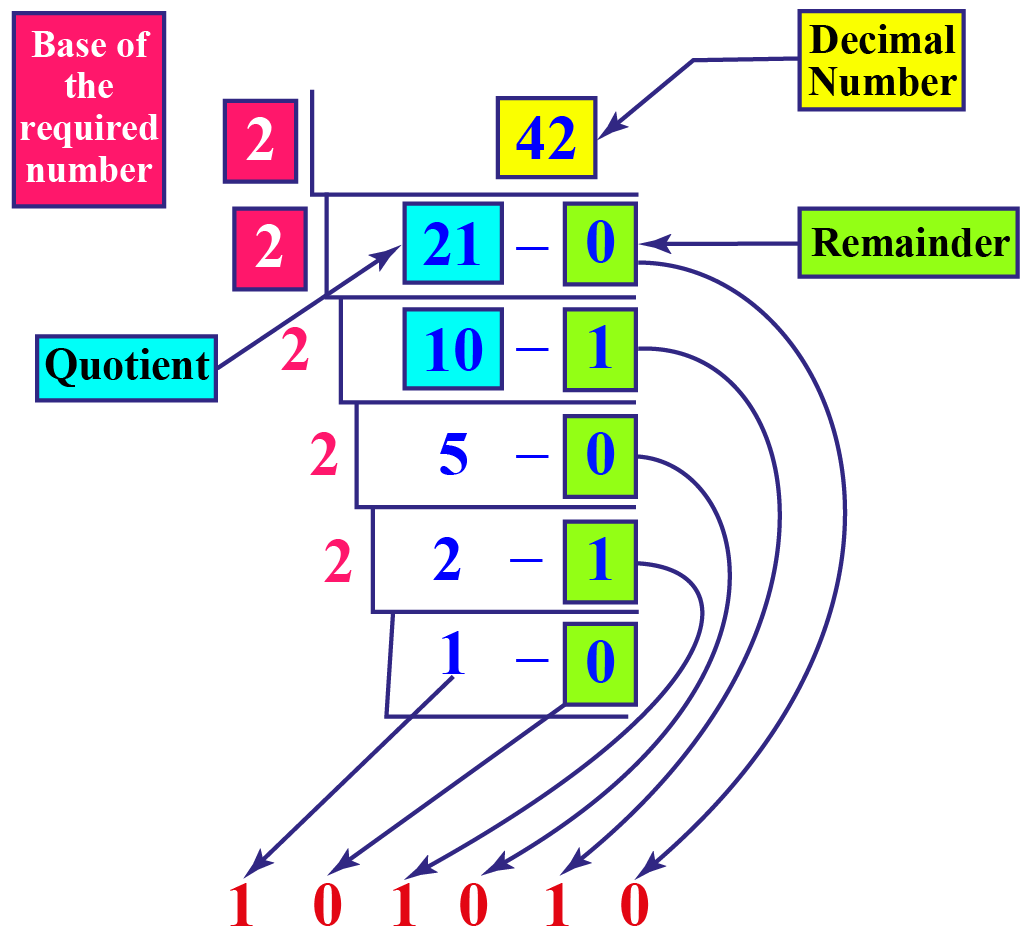Therefore  $${42}_{10} = {101010}_{2}$$

Convert 101010 From Binary to Decimal

What does 101010 mean?

The answer to the question can be found by converting $$101010_2$$ to decimal. So let's find out.

Step 1: Identify the base of the given number.

Here, the base of $$101010_2$$ is 2

Step 2: Multiply each digit of the given number starting from the rightmost digit, with the exponents of the base.

The exponents should always start with $$0$$ and increase by $$1$$ each time as we move from right to left.

Since the base here is $$2$$, we need to multiply the digits of the given number by $$2^0, 2^1, 2^2,...$$ from right to left.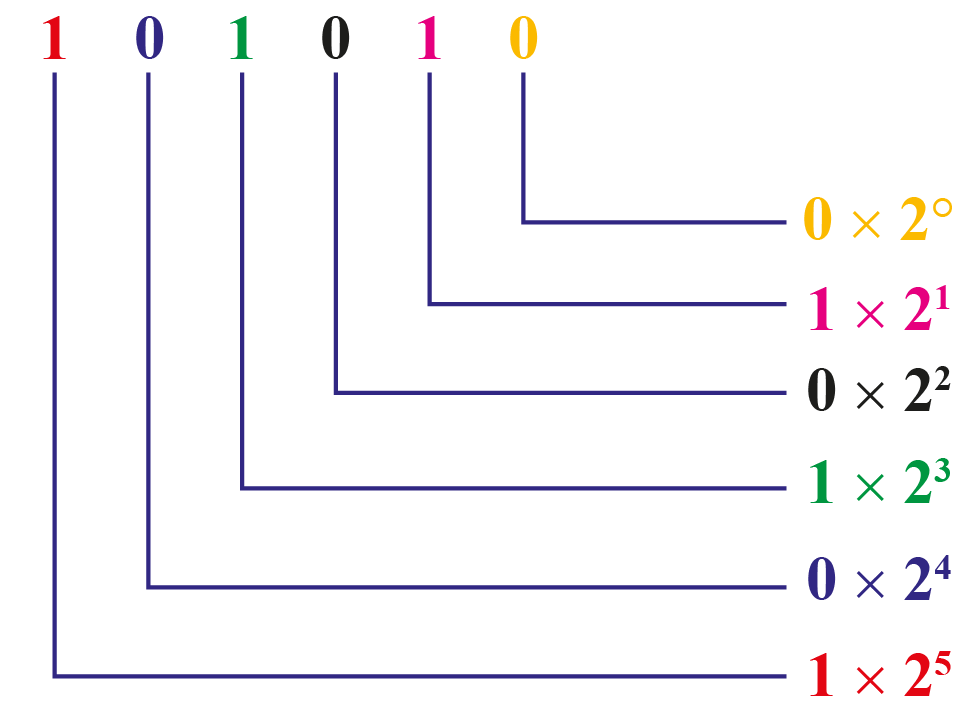Step 3: We just simplify each of the above products and add them.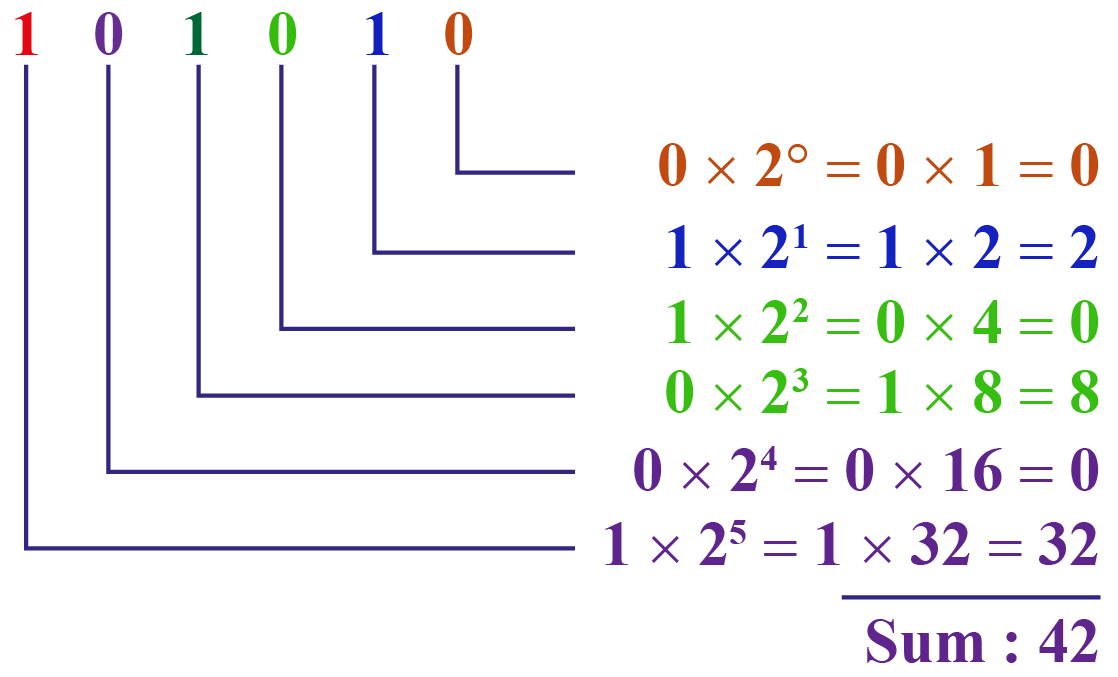This sum (39) is the decimal form of the number given in binary.

 $$\therefore 101010_2 = 42_{10}$$

More Important Topics
Numbers
Algebra
Geometry
Measurement
Money
Data
Trigonometry
Calculus